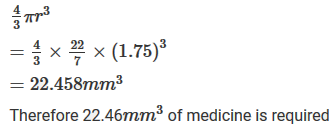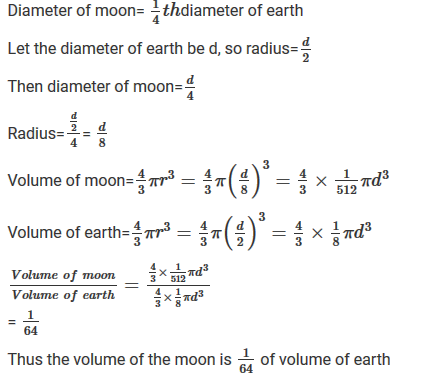#### Chapter 21 Surface Area and Volume of a Sphere R.D. Sharma Solutions for Class 9th Exercise 21.2

Exercise 21.2

1. Find the volume of a sphere whose radius is:

(i) 2 cm
(ii) 3.5 cm
(iii) 10.5 cm

Solution

Therefore volume=4/3πr3
= 4/3×22/7×(2)3
= 33.52cm3

Therefore volume=4/3πr3
= 4/3×22/7×(3.5)3 = 179.666cm3

Therefore volume=4/3πr3
= 4/3×22/7×(10.5)3 = 4851cm3

2. Find the volume of a sphere whose diameter is:
(i) 14 cm
(ii) 3.5 dm
(iii) 2.1 m

Solution

Therefore volume=4/3πr3
= 4/3×22/7×(7)3 = 1437.33cm3

Therefore volume=4/3πr3
=4/3×22/7×(1.75)3
=22.46dm3

(iii) Diameter = 2.1m, Radius(r)= 2.1/2 = 1.05m
Therefore volume=4/3πr3
= 4/3×22/7×(1.05)3 = 4.851m3

3. A hemispherical tank has the inner radius of 2.8 m. Find its capacity in liters.

Solution

Therefore Capacity= 2/3πr3
=2/3×22/7×(2.8)3 = 45.994m3
1m3=1000l
Therefore capacity in litres = 45994 litres .

4. A hemispherical bowl is made of steel 0.25 cm thick. The inside radius of the bowl is 5 cm. find the volume of steel used in making the bowl.

Solution

Outer radius = 5 + 0.25 = 5.25
Volume of steel used= Outer volume-Inner volume
= 2/3×π×((5.25)3− (5)3)
= 2/3×22/7×((5.25)3− (5)3)
= 41.282cm3

5. How many bullets can be made out of a cube of lead, whose edge measures 22 cm, each bullet being 2 cm in diameter ?

Solution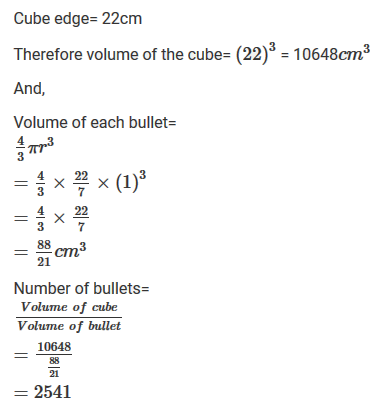Solution7. A spherical ball of lead 3 cm in diameter is melted and recast into three spherical balls. If the diameters of two balls be 3/2 cm and 2 cm, find the diameter of the third ball.

Solution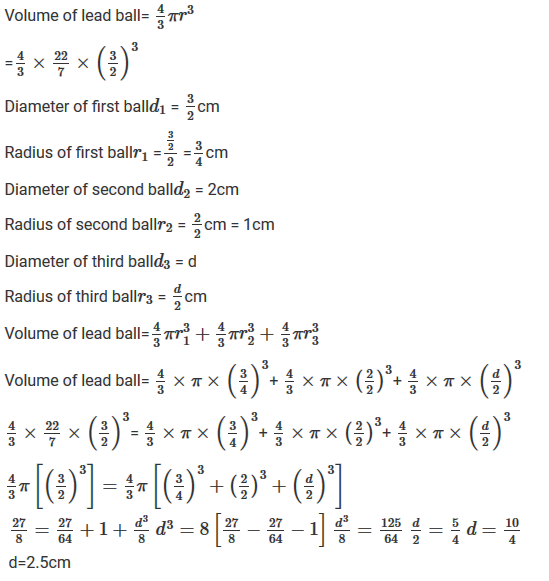8. A sphere of radius 5 cm is immersed in water filled in a cylinder, the level of water rises 5/3 cm. Find the radius of the cylinder.

Solution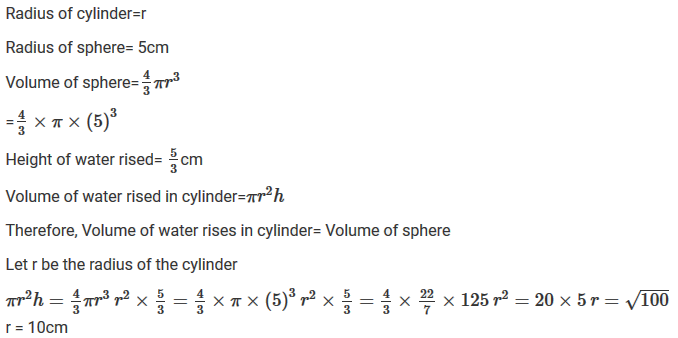9. If the radius of a sphere is doubled, what is the ratio of the volume of the first sphere to that of the second sphere ?

Solution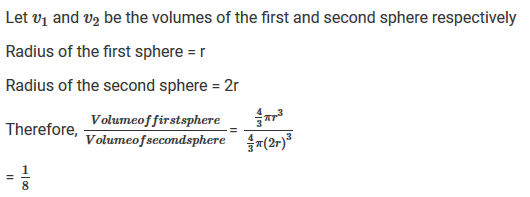10. A cone and a hemisphere have equal bases and equal volumes. Find the ratio of their heights.

Solution11. A vessel in the form of a hemispherical bowl is full of water. Its contents are emptied in a right circular cylinder. The internal radii of the bowl and the cylinder are 3.5 cm and 7 cm respectively. Find the height to which the water will rise in the cylinder.

Solution12. A cylinder whose height is two thirds of its diameter has the same volume as a sphere of radius 4 cm. Calculate the radius of the base of the cylinder.

Solution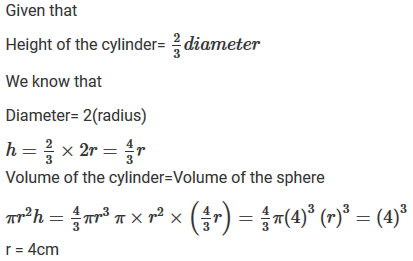13. A vessel in the form of a hemispherical bowl is full of water. The contents are emptied into a cylinder. The internal radii of the bowl and cylinder are respectively 6 cm and 4 cm. Find the height of water in the cylinder.

Solution14. A cylindrical tub of radius 16 cm contains water to a depth of 30 cm. A spherical iron ball is dropped into the tub and thus level of water is raised by 9 cm. What is the radius of the ball ?

Solution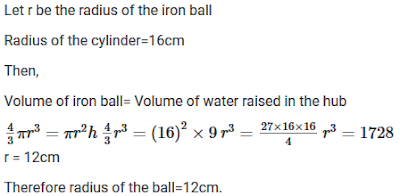15. A cylinder of radius 12 cm contains water to a depth of 20 cm. A spherical iron ball is dropped into the cylinder and thus the level of water is raised by 6.75 cm. Find the radius of the ball. (Use π =22/7).

Solution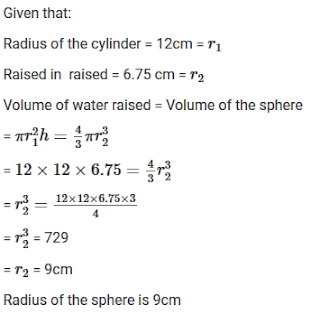16. The diameter of a coper sphere is 18 cm. The sphere is melted and is drawn into a long wire of uniform circular cross-section. If the length of the wire is 108 m, find its diameter.

Solution

Given that diameter of a copper sphere = 18cm
Radius of the sphere = 9cm
Length of the wire =108m =10800cm
Volume of cylinder = Volume of sphere17. A cylindrical jar of radius 6 cm contains oil. Iron spheres each of radius 1.5 cm are immersed in the oil. How many spheres are necessary to raise the level of the oil by two centimeters?

Solution
Given that,
Radius of the cylinder jar = 6cm = r1
Level to be rised = 2cm Radius of each iron sphere =1.5cm = r218. A measuring jar of internal diameter 10 cm is partially filled with water. Four equal spherical balls of diameter 2 cm each are dropped in it and they sink down in water completely. What will be the change in the level of water in the jar ?

Solution

Given that,
Diameter of jar = 10cm
Let the level of water be raised by h
Diameter of the spherical bowl = 2cm
Radius of the ball = 1cm
Volume of jar = 4(Volume of spherical ball)19. The diameter of a sphere is 6 cm. It is melted and drawn into a wire of diameter 0.2 cm. Find the length of the wire.

Solution20. The radius of the internal and external surfaces of a hollow spherical shell are 3 cm and 5 cm respectively. If it is melted and recast into a solid cylinder of height 22/3cm. Find the diameter of the cylinder.

Solution

Given that,
Internal radius of the sphere =3cm = r1
External radius of the sphere =5cm = r2
Height of the cylinder = 8/3cm = h
Volume of the spherical shell = Volume of cylinder .21. A hemisphere of the lead of radius 7 cm is cast into a right circular cone of height 49 cm. Find the radius of the base.

Solution

Given,
Radius of the hemisphere = Volume of cone22. A hollow sphere of internal and external radii 2cm and 4 cm respectively is melted into a cone of base radius 4cm. Find the height and slant height of the cone.

Solution

Given that
Hollow sphere external radii = r2 = 4cm
Height = h
Volume of cone = Volume of sphere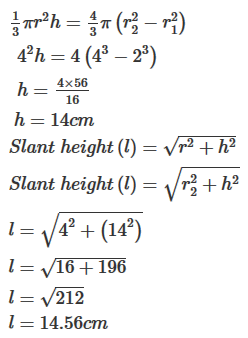23. A metallic sphere of radius 10.5 cm is melted and thus recast into small cones, each of radius 3.5 cm and height 3 cm. Find how many cones are obtained.

Solution

Given that,
Metallic sphere of radius = 10.5cm
Let the number of cones obtained be x24. A cone and a hemisphere have equal bases and equal volumes. Find the ratio of their heights.

Solution

Given that,
A cone and a hemisphere have equal bases and volumes25. A cone, a hemisphere, and a cylinder stand on equal bases and have the same height. Show that their volumes are in the ratio 1 : 2 : 3.

Solution
Given that
A cone, a hemisphere and a cylinder stand on one equal bases and have the same weight
We know that26. A cylindrical tub of radius 12 cm contains water to a depth of 20 cm. A spherical form ball is dropped into the tub and thus the level of water is raised by 6.75 cm. What is the radius of the ball?

Solution

Depth =20cm
Let r be the radius of the ball
Then
Volume of the ball= Volume of water raised27. The largest sphere is carved out of a cube of side 10.5 cm. Find the volume of the sphere.

Solution

Side of cube =10.5cm
Volume of sphere = v
Diameter of the largest sphere =10.5cm
2r = 10.5
r = 5.25cm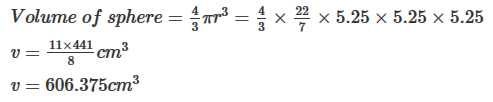28. A sphere, a cylinder, and a cone have the same diameter. The height of the cylinder and also the cone are equal to the diameter of the sphere. Find the ratio of their volumes.

Solution

Let r be the common radius
Height of the cone = height of the cylinder = 2r
Let29. A cube of side 4 cm contains a sphere touching its side. Find the volume of the gap in between.

Solution

It is given that
Cube side = 4cm
Volume of cube = (4cm)3 = 64cm3
Diameter of the sphere= Length of the side of the cube=4cm
Therefore radius of the sphere = 2cm .30. A hemispherical tank is made up of an iron sheet 1 cm thick. If the inner radius is 1 m, then find the volume of the iron used to make the tank.

Solution

Inner radius of the hemispherical tank = 1m = r1
Thickness of the hemispherical tank = 1cm = 0.01m
Outer radius of hemispherical tank = (1+0.01) = 1.01m =r2
Volume of iron used to make the tank =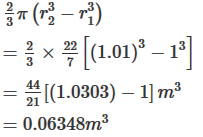31. A capsule of medicine is in the shape of a sphere of diameter 3.5 mm. How much medicine (mm3) is needed to fill this capsule?

Solution

Given that,
Diameter of capsule = 3.5mm